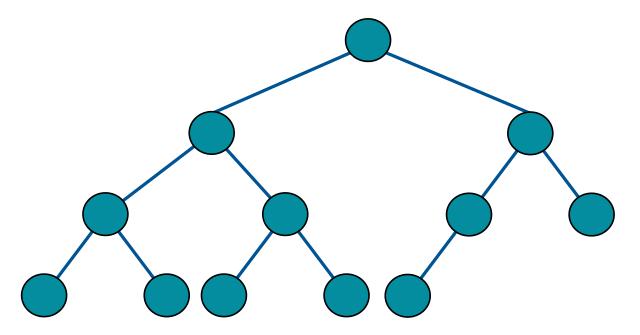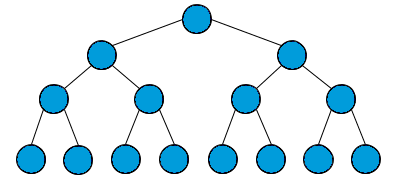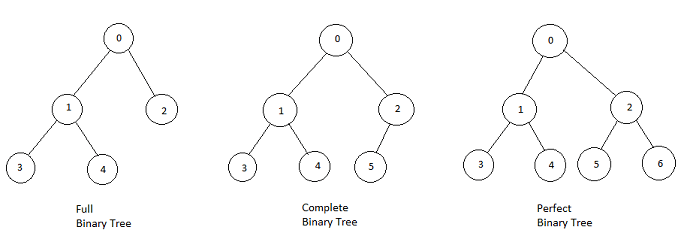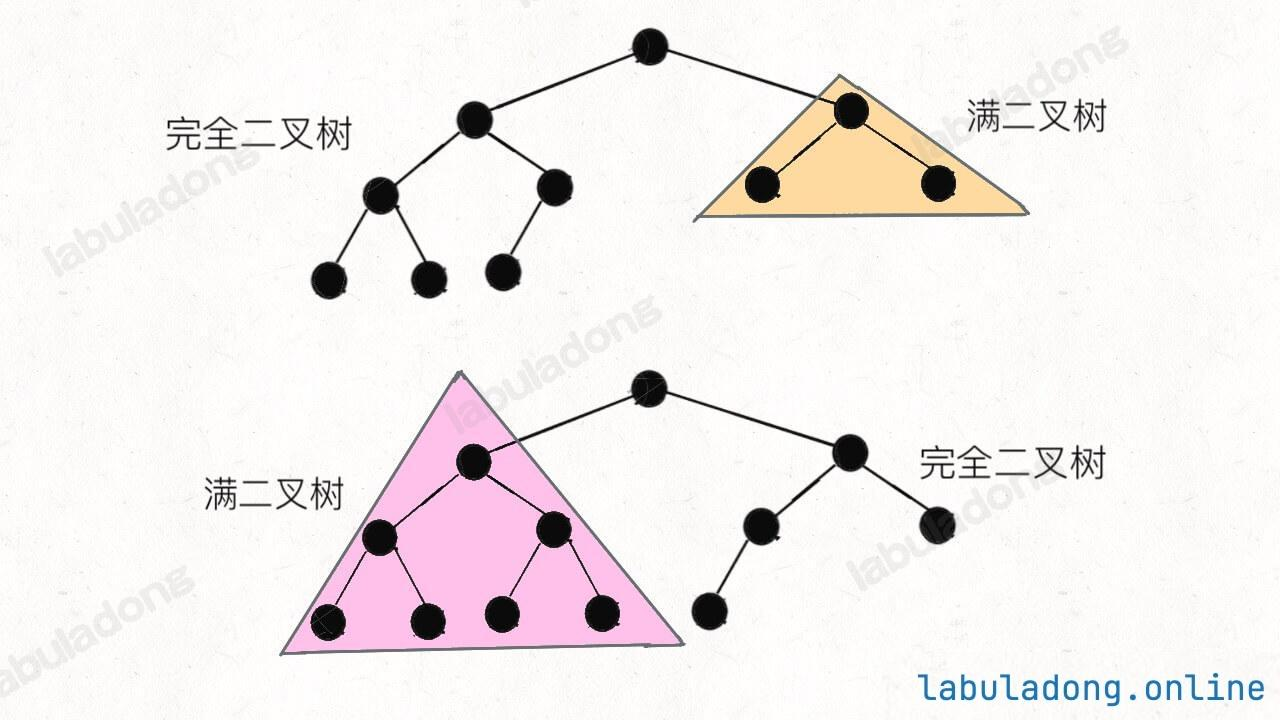Info

Info### # 一、思路分析

``````// 输入一棵完全二叉树，返回节点总数
int countNodes(TreeNode root);
``````

``````public int countNodes(TreeNode root) {
if (root == null) return 0;
return 1 + countNodes(root.left) + countNodes(root.right);
}
``````

``````public int countNodes(TreeNode root) {
int h = 0;
// 计算树的高度
while (root != null) {
root = root.left;
h++;
}
// 节点总数就是 2^h - 1
return (int)Math.pow(2, h) - 1;
}
``````

``````public int countNodes(TreeNode root) {
TreeNode l = root, r = root;
// 沿最左侧和最右侧分别计算高度
int hl = 0, hr = 0;
while (l != null) {
l = l.left;
hl++;
}
while (r != null) {
r = r.right;
hr++;
}
// 如果左右侧计算的高度相同，则是一棵满二叉树
if (hl == hr) {
return (int)Math.pow(2, hl) - 1;
}
// 如果左右侧的高度不同，则按照普通二叉树的逻辑计算
return 1 + countNodes(root.left) + countNodes(root.right);
}
``````

### # 二、复杂度分析

``````return 1 + countNodes(root.left) + countNodes(root.right);
``````